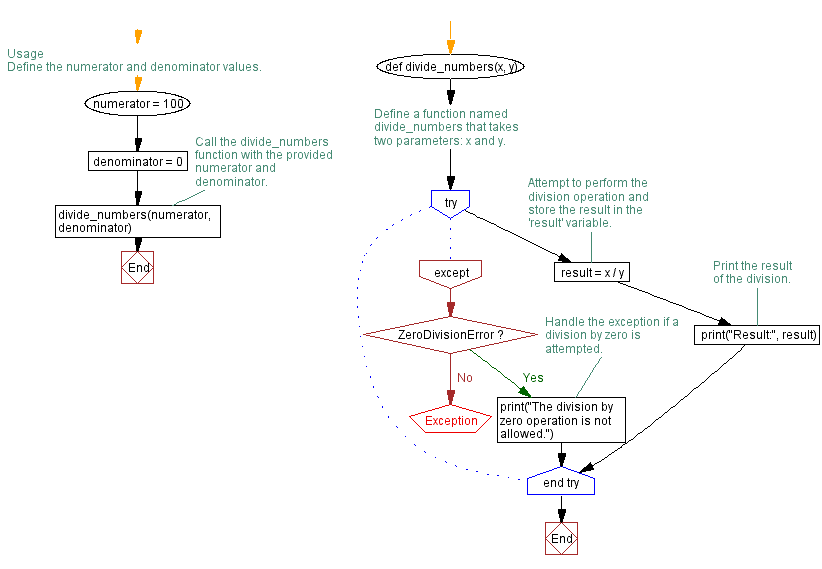﻿ Python ZeroDivisionError Exception Handling

# Python ZeroDivisionError Exception Handling

## Python Exception Handling: Exercise-1 with Solution

Write a Python program to handle a ZeroDivisionError exception when dividing a number by zero.

exception ZeroDivisionError:

Raised when the second argument of a division or modulo operation is zero. The associated value is a string indicating the type of the operands and the operation.

Sample Solution:

Code:

``````# Define a function named divide_numbers that takes two parameters: x and y.
def divide_numbers(x, y):
try:
# Attempt to perform the division operation and store the result in the 'result' variable.
result = x / y
# Print the result of the division.
print("Result:", result)
except ZeroDivisionError:
# Handle the exception if a division by zero is attempted.
print("The division by zero operation is not allowed.")

# Usage
# Define the numerator and denominator values.
numerator = 100
denominator = 0
# Call the divide_numbers function with the provided numerator and denominator.
divide_numbers(numerator, denominator)
``````

Explanation:

In the above exercise -

The "divide_numbers(0)" function takes two parameters, x and y, representing the numerator and denominator respectively. The try block within the function attempts to perform the division operation x / y.

If the division operation succeeds, the result is printed. However, if a ZeroDivisionError exception occurs because the denominator y is zero, the program jumps to the except block. The except block prints an error message indicating that division by zero is not permitted.

Output:

```The division by zero operation is not allowed.
```

Flowchart:What is the difficulty level of this exercise?

Test your Programming skills with w3resource's quiz.

﻿